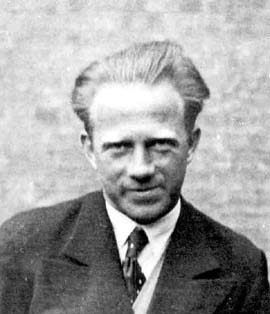A# uncertainty principleWerner Heisenberg, who first proposed the uncertainty principle in quantum mechanics.

The uncertainty principle is a principle of far-reaching importance in quantum mechanics, first proposed by Werner Heisenberg (and hence also known as the Heisenberg uncertainty principle) in 1927. It indicates the limit to the precision with which certain pairs of quantities connected with a particle, notably position and momentum, and time and energy, can be simultaneously known. The product of the uncertainties in each case must be greater than h/2π, where h is Planck's constant.

The uncertainty principle was arrived at from considerations of the dualistic nature of the electron. The very definition of a particle implies that at any instant it has a definite momentum and occupies a definite position in space. Unless we can simultaneously determine both the momentum and the position in space we can't actually say that a "particle" in the accepted sense has been observed. Since an electron is somewhere within the wave packet, moving with group velocity, uncertainty about the defined particle velocity arises, because the group is not infinitely narrow and has a velocity spread. It is impossible to know where, within the group, the electron actually is and what is its exact velocity. For a long wave packet, with many crests, the position of the electron is very uncertain but the velocity spread is very small so that the particle velocity is fairly accurately known. In a short packet the position of the particle is more or less fixed, but as the velocity spread of such a packet can be shown to be large, the particle velocity is indeterminate. Either the position or the velocity can be known accurately, but not both, and one has a doubtful value. It can be proved that it's impossible to determine simultaneously both the momentum and the position of a particle with accuracy. This is shown by the following treatment.

A wave packet representing a particle has a finite length Δx and the extreme ends have a wave number difference Δν. It can be shown from general wave theory Δx ~ 1/Δν, the length of the wave packet being inversely proportional to the difference in wave numbers of the two ends of the packet. Thus Δxν ~ 1. Since we have also λ = h/mv = h/p where the momentum mv is written as p, then

p = .

Differentiating gives

Δp/h = Δν

whence, by substitution, we get

Δxp ~ h.

It is clear that Δx represents the uncertainty in determining the exact location of the particle within the packet, and as there is a range of wave numbers Δν in the packet Δp is the uncertainty in evaluating the momentum. This then is Heisenberg's uncertainty relationship which states that the product of the uncertainties in determining position and momentum is approximately equal to Planck's constant h.

The more exactly we define the position, i.e., the smaller we make Δx, the larger becomes Δp and vice versa. The relationship shows that it is impossible to measure both the position and the momentum accurately simultaneously. Clearly this is fundamental since it sets a limit to the possible accuracy of observation. The uncertainty arises from the smeared-out properties of wave packets compared with finite points representing the centers of the particles.

No matter what method is tried it is impossible to avoid the consequences of the uncertainty relationship.